# plotPartialDependence

Create partial dependence plot (PDP) and individual conditional expectation (ICE) plots

## Syntax

``plotPartialDependence(Mdl,Vars)``
``plotPartialDependence(Mdl,Vars,X)``
``plotPartialDependence(___,Name,Value)``
``ax = plotPartialDependence(___)``

## Description

example

````plotPartialDependence(Mdl,Vars)` creates a PDP between features listed in `Vars` and responses predicted by using predictor data and a trained regression model in `Mdl`.```

example

````plotPartialDependence(Mdl,Vars,X)` creates a PDP by using new predictor data in `X`.```

example

````plotPartialDependence(___,Name,Value)` uses additional options specified by one or more name-value pair arguments in addition to any of the arguments in the previous syntaxes. For example, if you specify `'Conditional','absolute'`, the `plotPartialDependence` function creates a figure including a PDP, a scatter plot of the selected feature and predicted responses, and an ICE plot for each observation.```

example

````ax = plotPartialDependence(___)` returns the axes of the plot using any of the input arguments combinations in the previous syntaxes.```

## Examples

collapse all

Train a regression tree using the `carsmall` data set, and create a PDP that shows the relationship between a feature and the predicted responses in the trained regression tree.

Load the `carsmall` data set.

`load carsmall`

Specify `Weight`, `Cylinders`, and `Horsepower` as the predictor variables (`X`), and `MPG` as the response variable (`Y`).

```X = [Weight,Cylinders,Horsepower]; Y = MPG;```

Construct a regression tree using `X` and `Y`.

`Mdl = fitrtree(X,Y);`

View a graphical display of the trained regression tree.

`view(Mdl,'Mode','graph')`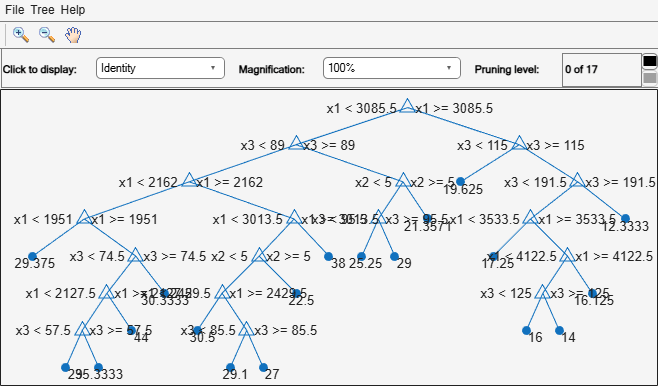Create a PDP of the first predictor variable, `Weight`.

`plotPartialDependence(Mdl,1)`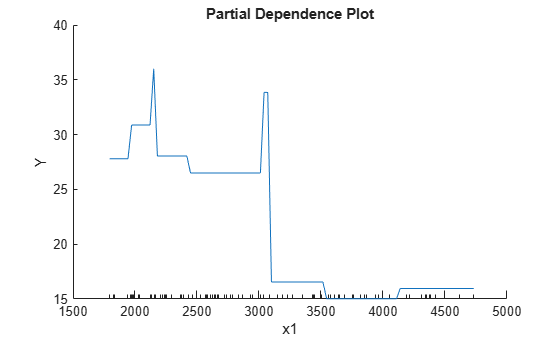The blue line represents averaged partial relationships between `Weight` (labeled as `x1`) and `MPG` (labeled as `Y`) in the trained regression tree `Mdl`.

The regression tree viewer shows that the first decision is whether `x1` is smaller than 3085.5. The PDP also shows a large change near `x1` = 3085.5. The tree viewer visualizes each decision at each node based on predictor variables. You can find several nodes split based on the values of `x1`, but it is not easy to figure out the dependence of `Y` on `x1`. However, the PDP plots averaged predicted responses against `x1`, so you can clearly see the partial dependence of `Y` on `x1`.

The labels `x1` and `Y` are the default values of the predictor names and the response name. You can modify these names by specifying the name-value pair arguments `'PredictorNames'` and `'ResponseName'` when you train `Mdl` using `fitrtree`. You can also modify axis labels by using the `xlabel` and `ylabel` functions.

Train a Gaussian process regression model using generated sample data where a response variable includes interactions between predictor variables. Then, create ICE plots that show the relationship between a feature and the predicted responses for each observation.

Generate sample predictor data `x1` and `x2`.

```rng('default') % For reproducibility n = 200; x1 = rand(n,1)*2-1; x2 = rand(n,1)*2-1;```

Generate response values that include interactions between `x1` and `x2`.

`Y = x1-2*x1.*(x2>0)+0.1*rand(n,1);`

Construct a Gaussian process regression model using `[x1 x2]` and `Y`.

`Mdl = fitrgp([x1 x2],Y);`

Create a figure including a PDP (red line) for the first predictor `x1`, a scatter plot (black circle markers) of `x1` and predicted responses, and a set of ICE plots (gray lines) by specifying `'Conditional'` as `'centered'`.

`plotPartialDependence(Mdl,1,'Conditional','centered')`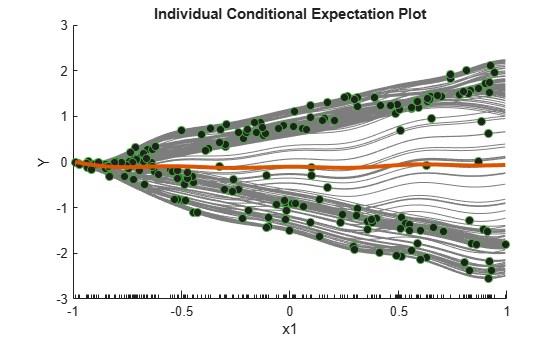When `'Conditional'` is `'centered'`, `plotPartialDependence` offsets plots so that all plots start from zero, which is helpful in examining the cumulative effect of the selected feature.

A PDP finds averaged relationships, so it does not reveal hidden dependencies especially when responses include interactions between features. However, the ICE plots clearly show two different dependencies of responses on `x1`.

Train a regression ensemble using the `carsmall` data set, and create a PDP plot and ICE plots for each predictor variable using a new data set, `carbig`. Then, compare the figures to analyze the importance of predictor variables. Also, compare the results with the estimates of predictor importance returned by the `predictorImportance` function.

Load the `carsmall` data set.

`load carsmall`

Specify `Weight`, `Cylinders`, `Horsepower`, and `Model_Year` as the predictor variables (`X`), and `MPG` as the response variable (`Y`).

```X = [Weight,Cylinders,Horsepower,Model_Year]; Y = MPG;```

Train a regression ensemble using `X` and `Y`.

```Mdl = fitrensemble(X,Y, ... 'PredictorNames',{'Weight','Cylinders','Horsepower','Model Year'}, ... 'ResponseName','MPG');```

Compare the importance of predictor variables by using the `plotPartialDependence` and `predictorImportance` functions. The `plotPartialDependence` function visualizes the relationships between a selected predictor and predicted responses. `predictorImportance` summarizes the importance of a predictor with a single value.

Create a figure including a PDP plot (red line) and ICE plots (gray lines) for each predictor by using `plotPartialDependence` and specifying `'Conditional','absolute'`. Each figure also includes a scatter plot (black circle markers) of the selected predictor and predicted responses. Also, load the `carbig` data set and use it as new predictor data, `Xnew`. When you provide `Xnew`, the `plotPartialDependence` function uses `Xnew` instead of the predictor data in `Mdl`.

```load carbig Xnew = [Weight,Cylinders,Horsepower,Model_Year]; f = figure; f.Position = [100 100 1.75*f.Position(3:4)]; % Enlarge figure for visibility. for i = 1 : 4 subplot(2,2,i) plotPartialDependence(Mdl,i,Xnew,'Conditional','absolute') end```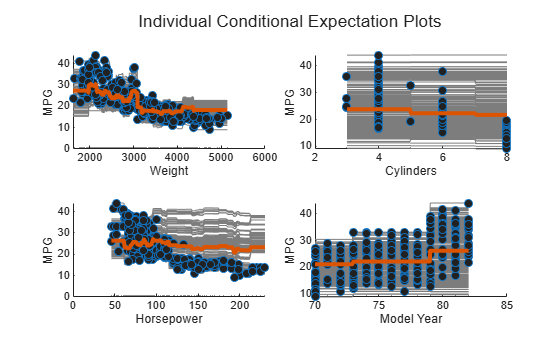Compute estimates of predictor importance by using `predictorImportance`. This function sums changes in the mean-squared error (MSE) due to splits on every predictor, and then divides the sum by the number of branch nodes.

```imp = predictorImportance(Mdl); figure; bar(imp); title('Predictor Importance Estimates'); ylabel('Estimates'); xlabel('Predictors'); ax = gca; ax.XTickLabel = Mdl.PredictorNames;```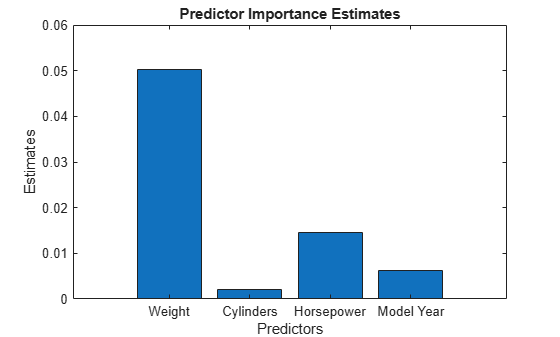The variable `Weight` has the most impact on `MPG` according to predictor importance. The PDP of `Weight` also shows that `MPG` has high partial dependence on `Weight`. The variable `Cylinders` has the least impact on `MPG` according to predictor importance. The PDP of `Cylinders` also shows that `MPG` does not change much depending on `Cylinders`.

Train a support vector machine (SVM) regression model using the `carsmall` data set, and create a PDP for two predictor variables. Then, extract partial dependence estimates from the output of `plotPartialDependence`.

Load the `carsmall` data set.

`load carsmall`

Specify `Weight`, `Cylinders`, and `Horsepower` as the predictor variables (`Tbl`).

`Tbl = table(Weight,Cylinders,Horsepower);`

Construct a support vector machine (SVM) regression model using `Tbl` and the response variable `MPG`. Use a Gaussian kernel function with an automatic kernel scale.

```Mdl = fitrsvm(Tbl,MPG,'ResponseName','MPG', ... 'KernelFunction','gaussian','KernelScale','auto');```

Create a PDP that visualizes partial dependence of predicted responses (`MPG`) on the predictor variables `Weight` and `Horsepower`. Use the `QueryPoints` name-value pair argument to specify the points to compute partial dependence.

```pt1 = linspace(min(Weight),max(Weight),50)'; pt2 = linspace(min(Horsepower),max(Horsepower),50)'; ax = plotPartialDependence(Mdl,{'Weight','Horsepower'},'QueryPoints',[pt1 pt2]); view(140,30) % Modify the viewing angle```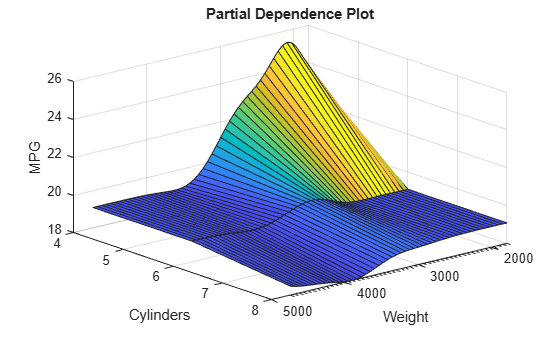The PDP shows an interaction effect between `Weight` and `Horsepower`.

Extract the estimated partial dependence of `MPG` on `Weight` and `Horsepower`. The `XData`, `YData`, and `ZData` values of `ax.Children` are x-axis values (the first selected predictor values), y-axis values (the second selected predictor values), and z-axis values (the corresponding partial dependence values), respectively.

```xval = ax.Children.XData; yval = ax.Children.YData; zval = ax.Children.ZData;```

If you specify `'Conditional'` as `'absolute'`, `plotPartialDependence` creates a figure including a PDP, a scatter plot, and a set of ICE plots. `ax.Children(1)` and `ax.Children(2)` correspond to the PDP and scatter plot, respectively. The remaining elements of `ax.Children` correspond to the ICE plots. The `XData` and `YData` values of `ax.Children(i)` are x-axis values (the selected predictor values) and y-axis values (the corresponding partial dependence values), respectively.

## Input Arguments

collapse all

Trained regression model, specified as a full or compact regression model object described in the following table.

If `Mdl` is a compact regression model object, you must provide `X`.

`plotPartialDependence` does not support a trained model object with a sparse matrix. If you train `Mdl` by using `fitrlinear`, use a full numeric matrix for predictor data where rows correspond to individual observations.

Features to visualize, specified as a vector of positive integers, character vector or string scalar, string array, or cell array of character vectors. You can choose one or two features, as shown in the following tables.

One Feature

ValueDescription
positive integerIndex value corresponding to the column of the predictor data to visualize.
character vector or string scalar

Name of a predictor variable to visualize. The name must match the entry in `Mdl.PredictorNames`.

Two Features

ValueDescription
vector of two positive integersIndex values corresponding to the columns of the predictor data to visualize.
string array or cell array of character vectors

Names of the predictor variables to visualize. Each element in the array is the name of a predictor variable. The names must match the entries in `Mdl.PredictorNames`.

Example: `{'x1','x3'}`

Data Types: `single` | `double` | `char` | `string` | `cell`

Predictor data, specified as a numeric matrix or table. Each row of `X` corresponds to one observation, and each column corresponds to one variable.

• If `Mdl` is a full regression model object, `plotPartialDependence` uses the predictor data in `Mdl`. If you provide `X`, then `plotPartialDependence` does not use the predictor data in `Mdl` and uses `X` only.

• If `Mdl` is a compact regression model object, you must provide `X`.

`X` must be consistent with the predictor data that trained `Mdl`, stored in either `Mdl.X` or `Mdl.Variables`.

• If you trained `Mdl` using a numeric matrix, then `X` must be a numeric matrix. The variables making up the columns of `X` must have the same number and order as the predictor variables that trained `Mdl`.

• If you trained `Mdl` using a table (for example, `Tbl`), then `X` must be a table. All predictor variables in `X` must have the same variable names and data types as the names and types in `Tbl`. However, the column order of `X` does not need to correspond to the column order of `Tbl`.

• `plotPartialDependence` does not support a sparse matrix.

Data Types: `single` | `double` | `table`

### Name-Value Pair Arguments

Specify optional comma-separated pairs of `Name,Value` arguments. `Name` is the argument name and `Value` is the corresponding value. `Name` must appear inside quotes. You can specify several name and value pair arguments in any order as `Name1,Value1,...,NameN,ValueN`.

Example: `plotPartialDependence(Mdl,Vars,X,'NumObservationsToSample',100,'UseParallel',true)` creates a PDP by using 100 sampled observations in `X` and executing `for`-loop iterations in parallel.

Plot type, specified as the comma-separated pair consisting of `'Conditional'` and `'none'`, `'absolute'`, or `'centered'`.

ValueDescription
`'none'`

`plotPartialDependence` creates a PDP.

• If you select one numeric feature in `Vars`, `plotPartialDependence` computes partial dependence at values between the minimum and maximum of the selected feature and creates a PDP with a solid blue line.

• If you select one categorical feature in `Vars`, `plotPartialDependence` computes predicted responses at all categorical values and plots partial dependence with blue circle markers connected with a dotted line.

• If you select two features in `Vars`, `plotPartialDependence` creates a surface plot.

`'absolute'`

`plotPartialDependence` creates a figure including the following three types of plots:

• PDP with a solid red line

• Scatter plot of the selected feature and predicted responses with black circle markers

• ICE plot for each observation with a solid gray line

This value is valid when you select only one feature.

`'centered'`

`plotPartialDependence` creates a figure including the same three types of plots as `'absolute'`. `plotPartialDependence` offsets plots so that all plots start from zero.

This value is valid when you select only one feature.

For details, see Partial Dependence Plot and Individual Conditional Expectation Plots.

Example: `'Conditional','absolute'`

Number of observations to sample, specified as the comma-separated pair consisting of `'NumObservationsToSample'` and a positive integer. The default value is the number of total observations in either `Mdl` or `X`. If you specify a value larger than the number of total observations, then `plotPartialDependence` uses all observations.

`plotPartialDependence` samples observations without replacement by using the function `datasample` and uses the sampled observations to compute partial dependence. If you specify `'Conditional'` as either `'absolute'` or `'centered'`, `plotPartialDependence` creates a figure including an ICE plot for each sampled observation.

Example: `'NumObservationsToSample',100`

Data Types: `single` | `double`

Axes in which to plot, specified as the comma-separated pair consisting of `'ParentAxisHandle'` and an axes object. If you do not specify the axes and if the current axes are Cartesian, then `plotPartialDependence` uses the current axes (`gca`). If axes do not exist, `plotPartialDependence` plots in a new figure.

Example: `'ParentAxisHandle',ax`

Points to compute partial dependence, specified as the comma-separated pair consisting of `'QueryPoints'` and a numeric column vector, a numeric two-column matrix, or a cell array of two numeric column vectors.

The default value is a numeric column vector or a numeric two-column matrix, depending on the number of selected features, where a column contains 100 evenly spaced points between the minimum and maximum values of the selected feature.

• If you select one feature in `Vars`, use a numeric column vector.

• If you select two features in `Vars`:

• Use a numeric two-column matrix to specify the same number of points for each feature.

• Use a cell array of two numeric column vectors to specify a different number of points for each feature.

The default values for a categorical feature are all categorical values in the selected feature. You cannot modify `'QueryPoints'` for a categorical feature. If you select one numeric feature and one categorical feature, you can specify `'QueryPoints'` for a numeric feature by using a cell array consisting of a numeric column vector and an empty array.

Example: `'QueryPoints',{pt,[]}`

Data Types: `single` | `double` | `cell`

Flag to run in parallel, specified as the comma-separated pair consisting of `'UseParallel'` and `true` or `false`. If you specify `'UseParallel'` as `true`, `plotPartialDependence` executes `for`-loop iterations in parallel by using `parfor` when predicting responses for each observation and averaging them.

Example: `'UseParallel',true`

Data Types: `logical`

## Output Arguments

collapse all

Axes of the plot, returned as an axes object. For details on how to modify the appearance of the axes and extract data from plots, see Axes Appearance (MATLAB) and Extract Partial Dependence Estimates from Plots.

collapse all

### Partial Dependence Plot

A partial dependence plot (PDP) visualizes relationships between features and predicted responses in a trained regression model. `plotPartialDependence` creates either a line plot or a surface plot of predicted responses against a single feature or a pair of features, respectively, by marginalizing over the other variables.

Consider a PDP for a subset XS of the whole feature set X = {x1, x2, …, xm}. A subset XS includes either one feature or two features: XS = {xS1} or XS = {xS1, xS2}. Let XC be the complementary set of XS in X. A predicted response f(X) depends on all features in X:

f(X) = f(XS, XC).

The partial dependence of predicted responses on XS is defined by the expectation of predicted responses with respect to XC:

`${f}^{S}\left({X}^{S}\right)={E}_{C}\left[f\left({X}^{S},{X}^{C}\right)\right]=\int f\left({X}^{S},{X}^{C}\right){p}_{C}\left({X}^{C}\right)d{X}^{C},$`

where pC(XC) is the marginal probability of XC, that is, ${p}_{C}\left({X}^{C}\right)\approx \int p\left({X}^{S},{X}^{C}\right)d{X}^{S}$. Assuming that each observation is equally likely, and the dependence between XS and XC and the interactions of XS and XC in responses are not strong, `plotPartialDependence` estimates the partial dependence by using observed predictor data as follows:

 ${f}^{S}\left({X}^{S}\right)\approx \frac{1}{N}\sum _{i=1}^{N}f\left({X}^{S},{X}_{i}{}^{C}\right),$ (1)

where N is the number of observations and Xi = (XiS, XiC) is the ith observation.

`plotPartialDependence` creates a PDP by using Equation 1. Input a trained model (f(·)) and select features (XS) to visualize by using the input arguments `Mdl` and `Vars`, respectively. `plotPartialDependence` computes partial dependence at 100 evenly spaced points of XS or the points that you specify by using the `'QueryPoints'` name-value pair argument. You can specify the number (N) of observations to sample from given predictor data by using the `'NumObservationsToSample'` name-value pair argument.

### Individual Conditional Expectation Plots

An individual conditional expectation (ICE) plot, as an extension of a PDP, visualizes the relationship between a feature and the predicted responses for each observation. While a PDP visualizes the averaged relationship between features and predicted responses, a set of ICE plots disaggregates the averaged information and visualizes an individual dependence for each observation.

`plotPartialDependence` creates an ICE plot for each observation. A set of ICE plots is useful to investigate heterogeneities of partial dependence originating from different observations. `plotPartialDependence` can also create ICE plots with any predictor data provided through the input argument `X`. You can use this feature to explore predicted response space.

Consider an ICE plot for a selected feature xS with a given observation XiC, where XS = {xS}, XC is the complementary set of XS in the whole feature set X, and Xi = (XiS, XiC) is the ith observation. The ICE plot corresponds to the summand of the summation in Equation 1:

`${f}^{S}{}_{i}\left({X}^{S}\right)=f\left({X}^{S},{X}_{i}{}^{C}\right).$`

`plotPartialDependence` plots ${f}^{S}{}_{i}\left({X}^{S}\right)$ for each observation i when you specify `'Conditional'` as `'absolute'`. If you specify `'Conditional'` as `'centered'`, `plotPartialDependence` draws all plots after removing level effects due to different observations:

`${f}^{S}{}_{i,\text{centered}}\left({X}^{S}\right)=f\left({X}^{S},{X}_{i}{}^{C}\right)-f\left(\mathrm{min}\left({X}^{S}\right),{X}_{i}{}^{C}\right).$`

This subtraction ensures that each plot starts from zero, so that you can examine the cumulative effect of XS and the interactions between XS and XC.

### Weighted Traversal Algorithm

The weighted traversal algorithm is a method to estimate partial dependence for a tree-based regression model. The estimated partial dependence is the weighted average of response values corresponding to the leaf nodes visited during the tree traversal.

Let XS be a subset of the whole feature set X and XC be the complementary set of XS in X. For each XS value to compute partial dependence, the algorithm traverses a tree from the root (beginning) node down to leaf (terminal) nodes and finds the weights of leaf nodes. The traversal starts by assigning a weight value of one at the root node. If a node splits by XS, the algorithm traverses to the appropriate child node depending on the XS value. The weight of the child node becomes the same value as its parent node. If a node splits by XC, the algorithm traverses to both child nodes. The weight of each child node becomes a value of its parent node multiplied by the fraction of observations corresponding to each child node. After completing the tree traversal, the algorithm computes the weighted average by using the assigned weights.

For an ensemble of regression trees, the estimated partial dependence is an average of the weighted averages over the individual regression trees.

## Algorithms

`plotPartialDependence` uses a `predict` function to predict responses. `plotPartialDependence` chooses the proper `predict` function according to `Mdl` and runs `predict` with its default settings. For details about each `predict` function, see the `predict` function in the following table. If `Mdl` is a tree-based model (not including a boosted ensemble of trees) and `'Conditional'` is `'none'`, then `plotPartialDependence` uses the weighted traversal algorithm instead of the `predict` function. For details, see Weighted Traversal Algorithm.

 Friedman, J. H. “Greedy function approximation: a gradient boosting machine.” The Annals of Statistics. Vol. 29, No. 5, 2001, pp. 1189-1232.

 Goldstein, A., A. Kapelner, J. Bleich, and E. Pitkin. “Peeking inside the black box: Visualizing statistical learning with plots of individual conditional expectation.” Journal of Computational and Graphical Statistics. Vol. 24, No. 1, 2015, pp. 44-65.

 Hastie, T., R. Tibshirani, and J. H. Friedman. The Elements of Statistical Learning. New York: Springer, 2001.